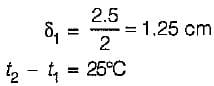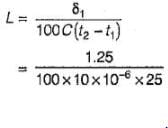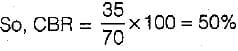# Test: Pavement Design - 2

## 20 Questions MCQ Test Topicwise Question Bank for Civil Engineering | Test: Pavement Design - 2

Description
Attempt Test: Pavement Design - 2 | 20 questions in 60 minutes | Mock test for Civil Engineering (CE) preparation | Free important questions MCQ to study Topicwise Question Bank for Civil Engineering for Civil Engineering (CE) Exam | Download free PDF with solutions
QUESTION: 1

### The width of expansion joint gap is 2.5 cm in a cement concrete pavement. The spacing between expansion joint for a maximum rise in temperature of 25°C is (assuming coefficient of thermal expansion of concrete as 10 x 10-6 per °C)

Solution:Coefficient of thermal expansion
C = 10 X 10-6 per°C
∴ Spacing of expansion joint,= 50m

QUESTION: 2

Solution:
QUESTION: 3

### Base course is used in rigid pavements for

Solution:
QUESTION: 4

The standard plate size in a plate bearing test for finding modulus of sub-grade reaction (k) value is

Solution:
QUESTION: 5

Consider the following statements:
1. In a concrete pavement temperature stress is tensile at bottom during day time.
2. In a concrete pavement load stress is compressive at bottom.
Which of these statements is/are correct?

Solution:
QUESTION: 6

It is customary to design the thickness of cement concrete pavement for

Solution:

The wheel load stress for rigid pavements are computed at interior, edge and corner using Westergaard’s equation. Temperature, stress are developed in cement concrete pavements leading to warping and frictional stress. Critical combination of stress (load and temperature) is evaluated to determine the thickness of cement concrete pavement.

QUESTION: 7

In reinforced cement concrete pavements, steel is placed

Solution:

The reinforcement used in cement concrete pavement is in the form of welded wire fabric or bar mats. The function of reinforcement is to hold the cracked slab portions together and thus not to allow them to open up any more. In order to obtain the maximum advantage, it is suggested that greater quantity of the reinforcement should be placed in the longitudinal direction. Further the reinforcement should either be placed in the middepth or towards the top of the pavement for better functioning.

QUESTION: 8

In cement concrete pavements, tie bars are provided:

Solution:

Tie bar are not the load transferring devices. These are provided to ensure the adjacent slabs remain firmly tied together. These are provided in the transverse direction across longitudinal joints, (warping joint) at mid depth.

QUESTION: 9

The sub-grade soil properties of a sam ple are as follows:
Soil portion passing 0.075 mm sieve = 50%
Liquid limit = 40%
Plasticity index = 20%
The group index of the soil is

Solution:

Group Index
Gl = 0.2 a + 0.005 ac + 0,01 bd
Soil portion passing 0.075mm sieve
P = 50%
LL - 40% PI = 20%
a = P - 3 5 - 15 < 40
b = P - 1 5 = 35 < 4 0
c = LL - 40 = 0
d = PI - 10 = 10
G.I. = 0.2 x 15 + 0 + 0.01 x 35 x 10
= 3 + 3.5 = 6,5

QUESTION: 10

Consider the following factors :
2. Thickness of cement concrete slab
3. Temperature distribution in the slab
Which of these should be taken into reckoning to determine the wheel load stress at critical location in a cement concrete pavement?

Solution:

To determine wheel load'stress at critical location, - Magnitude of load (P) - Thickness of slab (h) - Radius of wheel Load distribution (a), radius of relative stiffness (I) and radius of resisting section (b) are needed. 'a', 'b' and 'I’ depend upon modulus of sub - grade reaction.

QUESTION: 11

If the pressure carried by a CBR specim en at 2.5 mm penetration is 3.5 N/mm2, the CBR of the soil is

Solution:

The pressure 3.5 N/mm2 = 35 kg/cm2QUESTION: 12

Consider the following statements: The failure of sub-grade of a flexible pavement is mainly attributed to
2. loss of binding action
3. loss of base course materials
4. excessive stress concentration
Which of the statements are correct?

Solution:

Two basic reasons for failure of sub-grade are:
(ii) Excessive stress application.

QUESTION: 13

Consider the following statements: In the design of modern concrete pavements, tie bars are used:
2. In expansion joints
3. In contraction joints
4. In warping joints
Which of the statements given above is/are correct?

Solution:

Tie bars are used across longitudinal joints (warping joints). They are not used a load transferring devices. Tie bars are thus designed to with stand tensile stresses, and the maximum tensile force in tie bars being equal to the force required to overcome frictional force between the bottom of the adjoining pavement slab and the soil sub-grade. Dowei bars are provided at expansion and contraction joints and they act as load transferring devices.

QUESTION: 14

If the CBR value obtained at 5 mm penetration is higher than that at 2.5 mm, then the test is repeated for checking: and if the check test reveals a. similar trend, then the CBR value is to be reported as the

Solution:

The average CBR value of three test specimens is reported to the first decimal place, as the GBR value of the test specimen. If the maximum variation' iii the CBR values of these specimens exceeds specified limits (3% for CB  up to 10% to 5% for CBR 10 to 30% and 10% for CBR 30-60%), then the design CBR should be the average of at least six specimens.

QUESTION: 15

If the load, warping and frictional stresses in a cement concrete slab are 210 N/mm2, 290 N/mm2 and 10 N/mm2 respectively, the critical combination of stresses during summer midday is

Solution:

Critical combination of stresses:
(i) During Summer mid-day at bottom of slab (Load stress + warpings tress - friction stress), at edge region = 210 + 290 - 10 = 490 N/mm2
(ii) During winter mid -day at bottom of slab
(Load stress + warping stress + friction stress); at edge region = 210 + 290 + 10 = 510N/mm2
(iii) At top Of slab during midnights
(Load stress + warping stress), at corner region.

QUESTION: 16

In the design of highway expansion and contraction joints should respectively be provided at

Solution:
QUESTION: 17

In the CBR design method recommended by the IRC for the design of flexible pavement, the total thickness depends upon

Solution:
QUESTION: 18

If the radius of wheel load distribution is 30 cm and slab thickness is 15 cm, then the equivalent radius of resisting section is

Solution:

Because the radius of wheel load distribution is more than 1.724 times slab thickness, hence radius of resisting section is equal to the radius of wheel load distribution.

QUESTION: 19

The critical combination of stresses for corner region in cement concrete roads is

Solution:
QUESTION: 20

Group index method of design of flexible pavement is

Solution:

The Gl method of pavement design is essentially an empirical method based on physical properties of the sub-grade soil. This method does- not consider the strength characteristic of the subgrade soil.Use Code STAYHOME200 and get INR 200 additional OFF Use Coupon Code